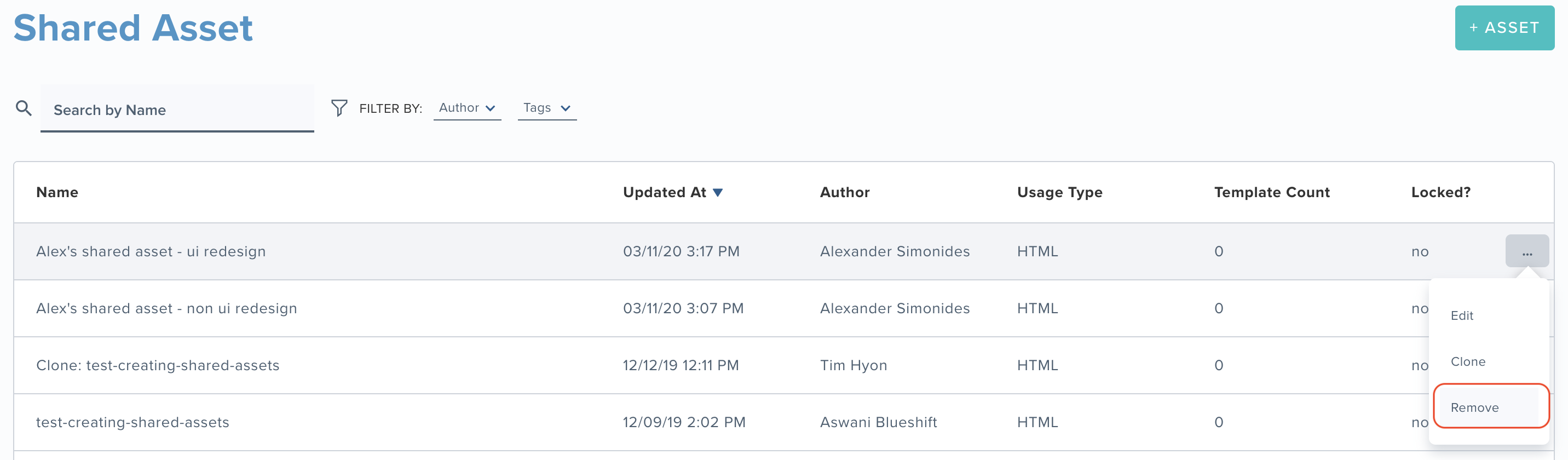# Nested shared assets

Shared assets provide a convenient way to apply consistent content such as headers, footers, product grids, and promotion banners across multiple templates by reusing snippets of code.

To keep your email code clean, you can combine shared assets by including one shared asset in another shared asset. This is referred to as nesting. The shared asset that is used in another shared asset is called a ‘nested shared asset’.

Consider the following points when nesting shared assets:

• You can only use a shared asset of the same type inside another shared asset. For example, a shared asset template of type HTML can include another shared asset template of type HTML only.
• You can nest shared assets up to 1 level. For example,
• Shared asset A does not reference any other assets. Shared asset B references shared asset A. Hence the nesting depth is 1 (B ←A), which is acceptable.
• If we have shared asset C, it cannot reference shared asset B as the depth would become 2. (C← B ← A).
• However, shared asset C can reference shared asset A (C ←A). In other words, it is possible to reference a shared asset in multiple shared assets.
• The Templates using this asset section of the shared asset displays all the other shared assets that include this shared asset along with email templates that use the shared asset.

Example: Shared asset A does not reference any other assets. Share asset B references shared asset A. Hence the depth is 1 (B ← A).

Shared Asset A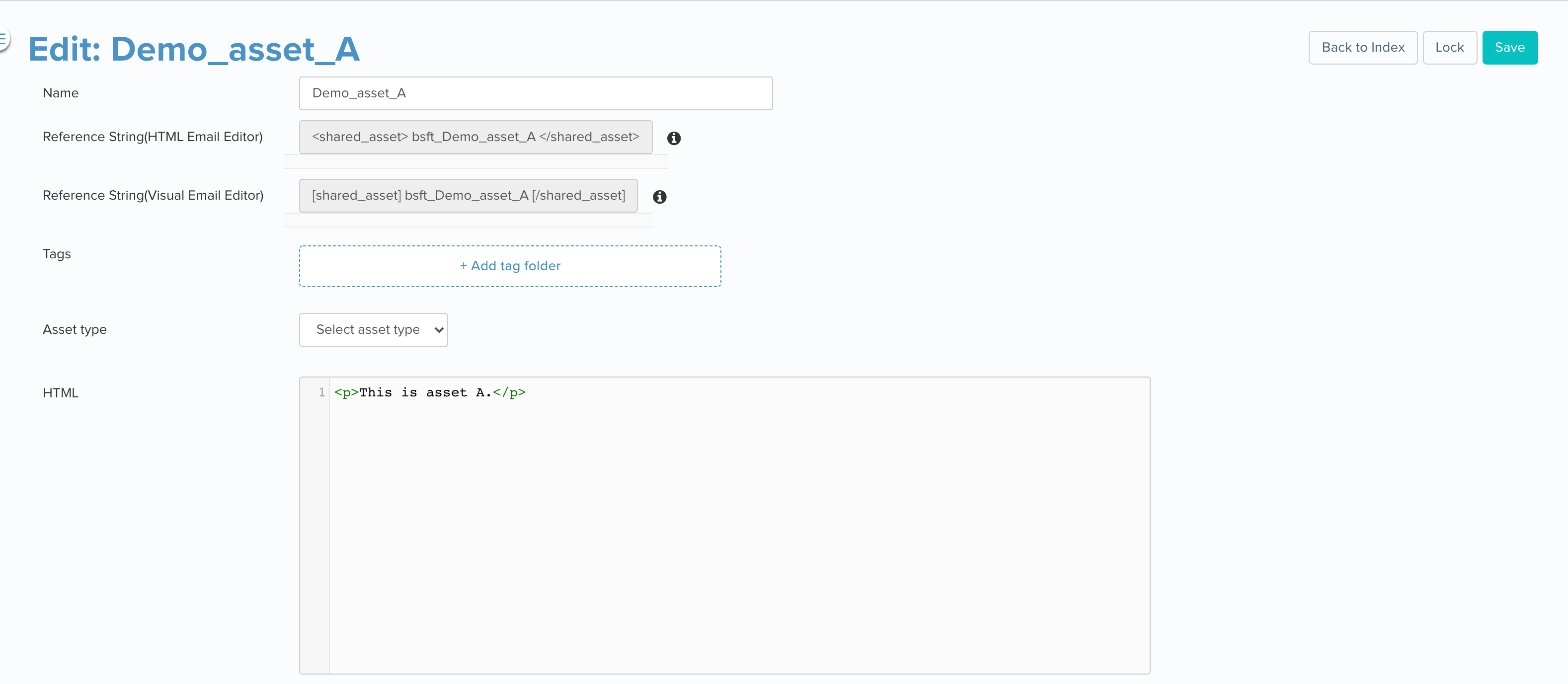Shared Asset B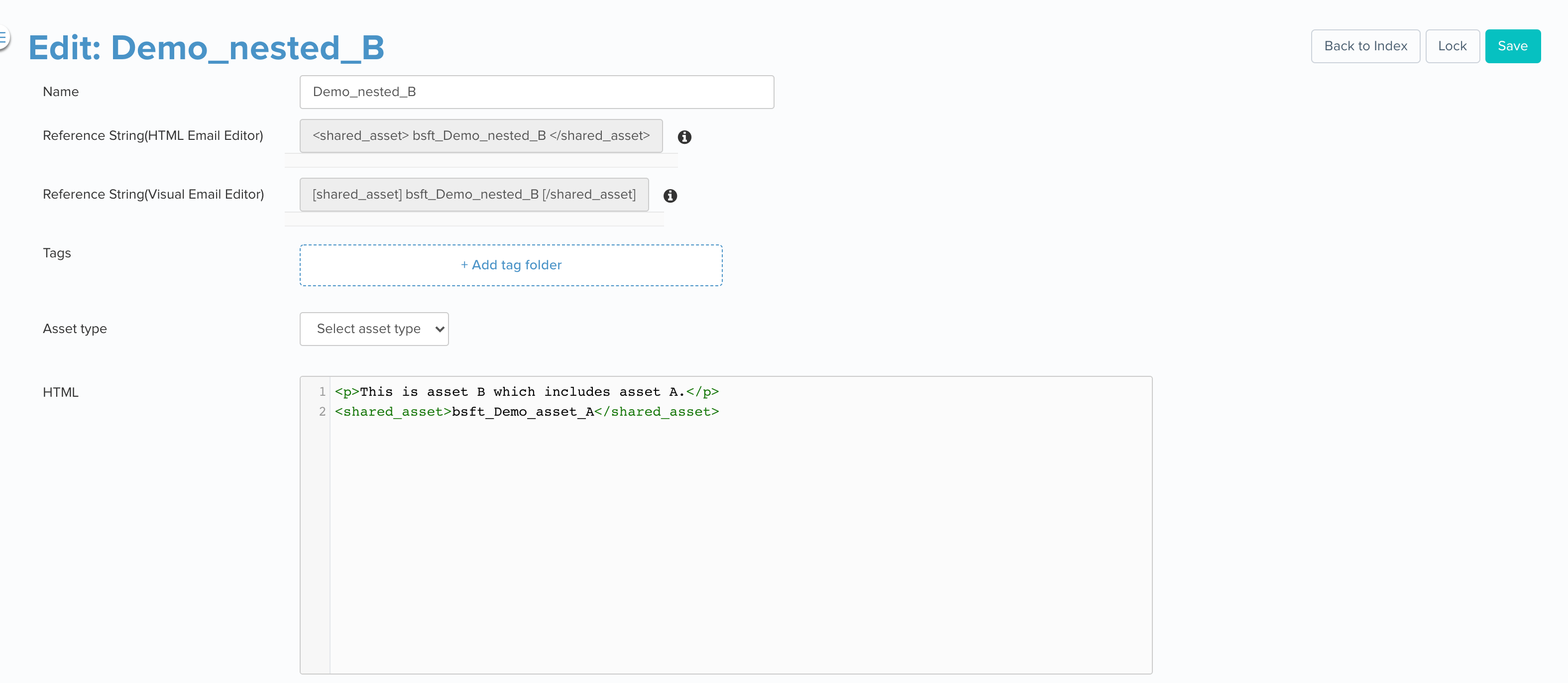## Syntax for nested shared assets

The syntax for nesting a shared asset in another shared asset depends on where the nested asset will be used. Since the reference string syntax for using a shared asset in an HTML template is different from the syntax for using a shared asset in a Visual Editor template, you must be careful when you combine assets.

Example:

Consider shared asset A which does not reference any other assets. We want shared asset B to reference (include) shared asset A.

Visual editor: If you are going to use shared asset B in a Visual Editor email template, use the syntax [shared_asset]bsft_Demo_asset_A[/shared_asset].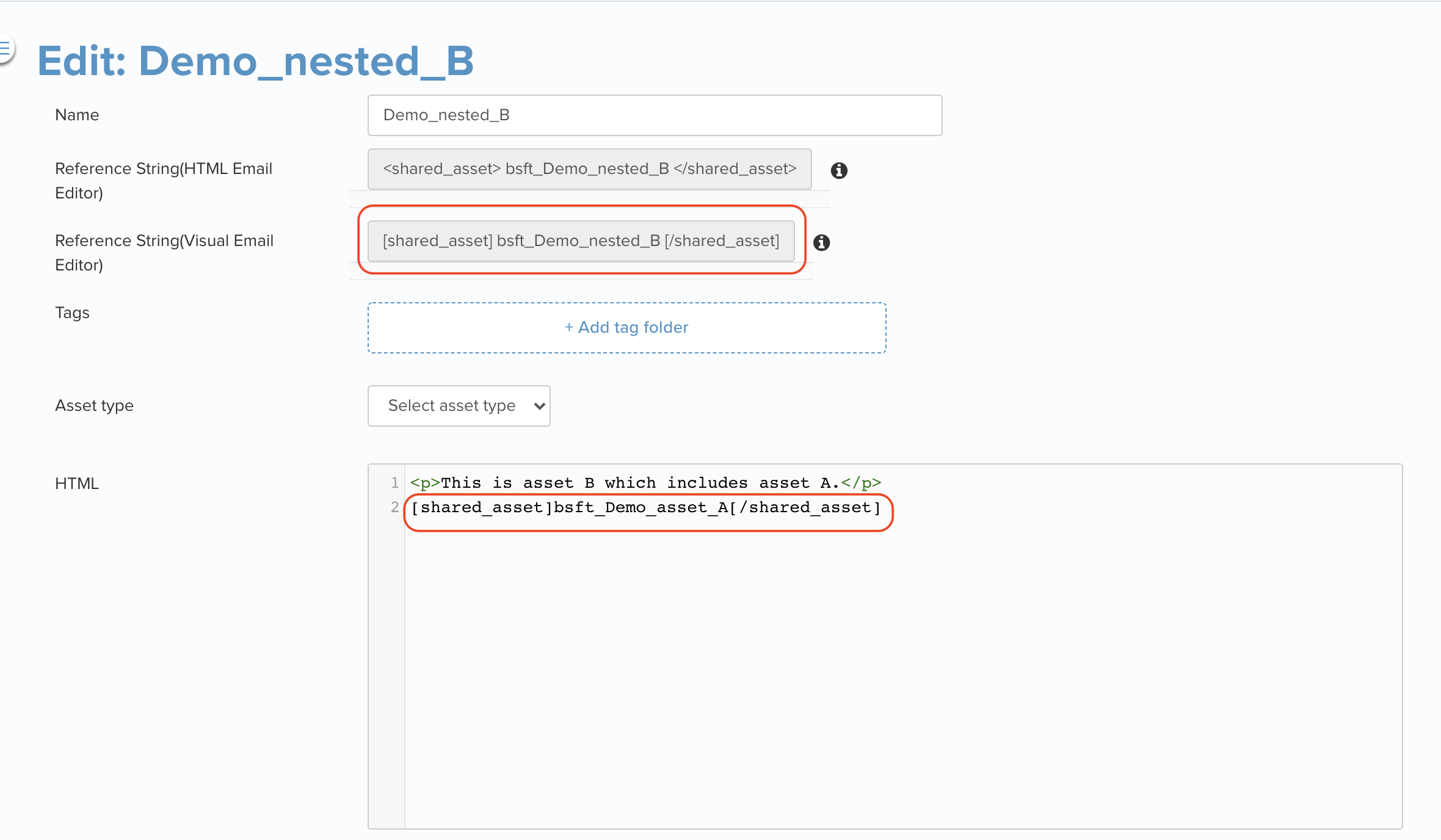HTML Editor: If shared asset B is to be used in an HTML Editor email template, use <shared_asset>bsft_Demo_asset_A</shared_asset>.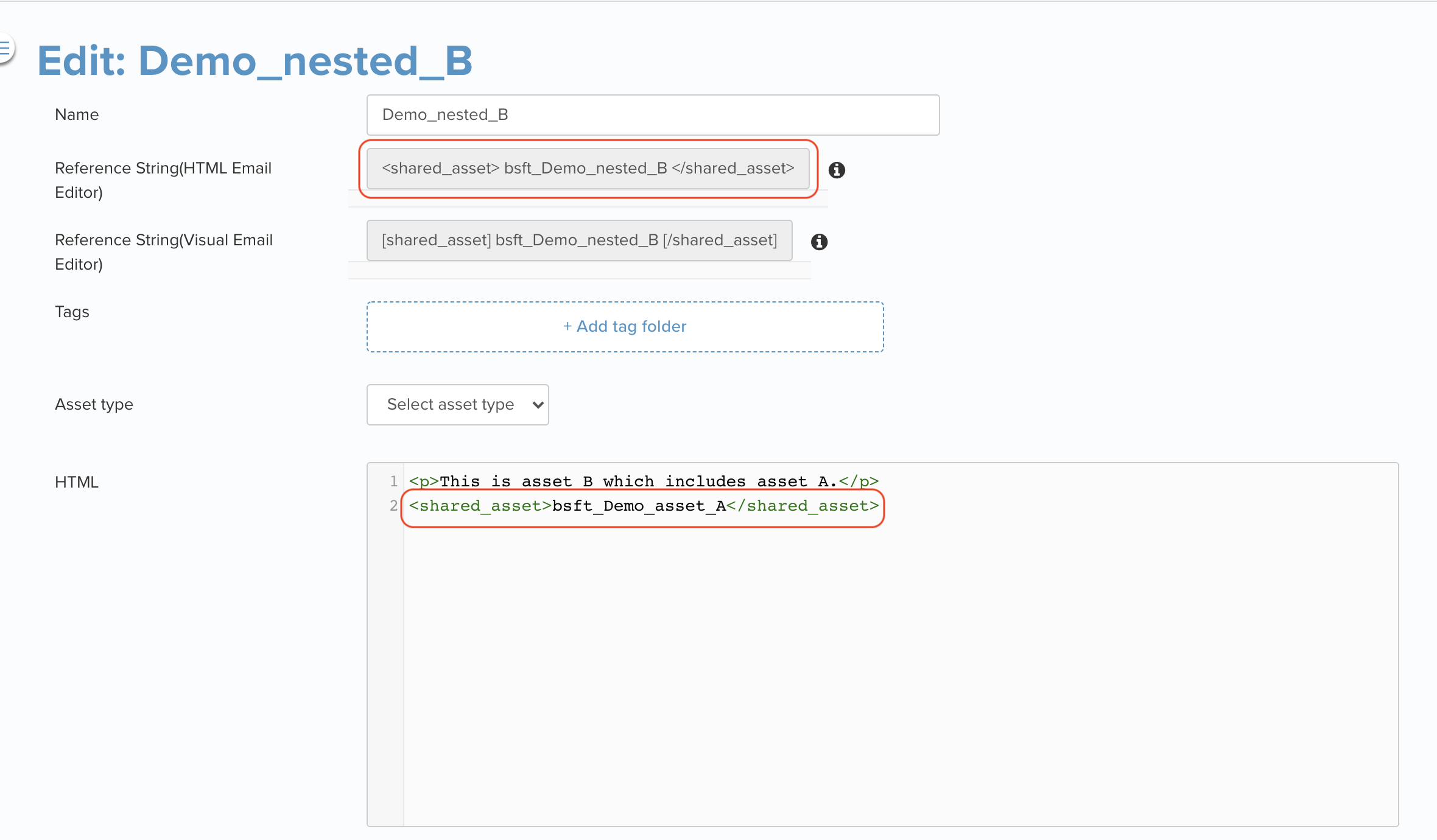## Lock nested assets

• When you lock an asset that contains nested assets, the nested assets are also locked. For example, if shared asset B uses shared asset A (i.e. asset A nested under asset B), locking shared asset B will also lock shared asset A.
• Similarly, when you unlock a parent asset, all child assets are unlocked. If both assets B and A are locked, then unlocking asset A will also unlock asset B.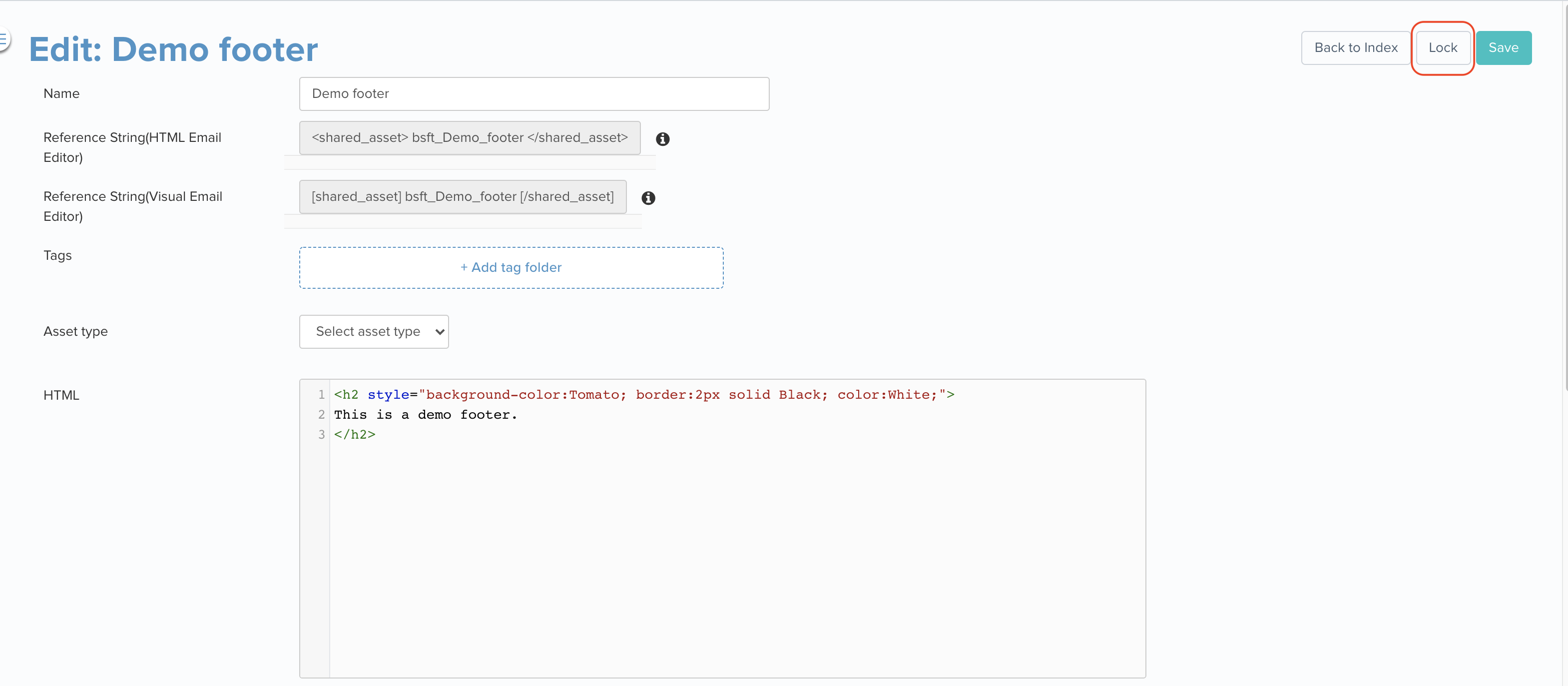## Delete an asset

• Use the Remove option on the shared asset listing page to delete a shared asset.
• A shared asset cannot be deleted if it is being referenced in any templates or shared assets.